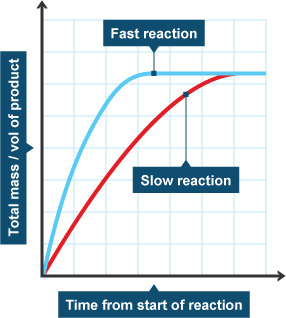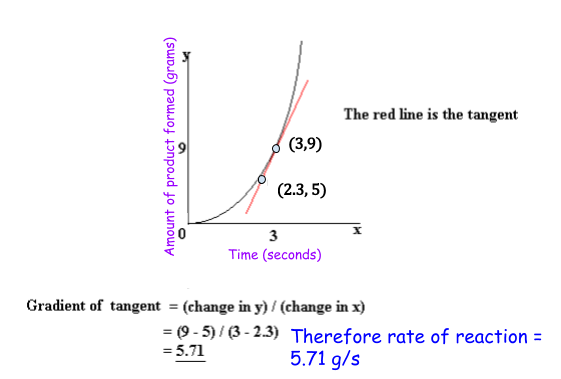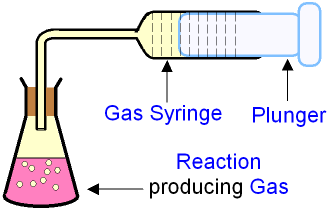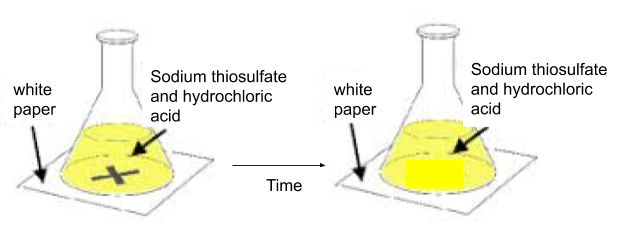# Analysis of Rates of Reaction

## Rate Graphs 1

What you need to know:

How to calculate the reaction rate by finding the gradient of a reaction-time graph.There are two main ways to find the rate of a reaction, using the equation __above or finding the __gradient of a reaction rate graph.The gradient __(‘slope’) of the graph tells you how __fast the reaction is. The steeper the gradient, the faster the reaction! For this to work you need to make sure that time is on the x-axis.

Since the reaction rate is changing all the time, we need to pick a time to work out the reaction rate.

__Here is how to find the gradient (and therefore reaction rate): __

1. Draw a__ tangent__ to the curve of where you want to find that rate of reaction.
2. __Pick two points __on that tangent line.
3. Work out the__ difference in the y-coordinates__ of the two points you picked.
4. Work out the difference in the x-coordinates of the two points you picked.
5. Divide the difference in y by the difference in x.
6. This gives you the gradient, which is the rate of the reaction!

## Rate Graphs 2

Here is how to find the gradient (and therefore reaction rate):

1. Draw a tangent to the curve of where you want to find that rate of reaction.
2. Pick two points on that tangent line.
3. Work out the difference in the y-coordinates of the two points you picked.
4. Work out the difference in the x-coordinates of the two points you picked.
5. Divide the difference in y by the difference in x.
6. This gives you the gradient, which is the rate of the reaction!

Worked example:## Reaction Rate Expeiments

What you need to know:

How to find the rate of reaction of the two experiments below.

You will need to know two experiments and how to measure their rates of reaction:

Magnesium and hydrochloric acid:As you can see, you are reacting a solid with a liquid to produce a liquid and a gas.

You can measure the rate of reaction by collecting the gas in a syringe and measuring the volume of gas that is produced.Sodium thiosulfate and hydrochloric acid:As you can see, you are reacting a liquid with a liquid to produce __a liquid, a gas, a solid and a liquid. __

You can measure the rate of reaction by timing how long it takes for the solution to become opaque (can’t see through it). You can do this by placing the flask on a piece of paper with an x drawn on. You can time how long it takes until you can no longer see the x.